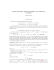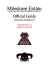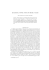# Homework 3

## Transcription

Homework 3
```ICS 6D
Due: Wednesday, October 29, 2014, 3:00PM
Homework 3
Instructor: Sandy Irani
1. Prove that any postage of 8 cents or more can be made from 5-cent or 3-cent stamps.
2. Problem 1 from homework 2 ask you to define the set of properly nested sequences that have parentheses and curly braces. Give an inductive proof that for sequences in this set, the number of left
regular parens ( is the same as the number of right regular parens ) and the number of left curly braces
{ is equal to the number of right curly braces }.
3. The sequence {gn } is defined recursively as follows:
• g0 = 51
• g1 = 348.
• gn = 5 · gn−1 − 6 · gn−2 + 20 · 7n
Use induction to show that gn = 3n + 2n + 49 · 7n .
4.
• f0 =
• f1 =
5
3
11
3 .
• fn = 3 · fn−1 + 4 · fn−2 + 6n
Use induction to show that fn = 2 · 4n + 32 (−1)n − n −
11
6 .
5. Compute the value of the following expressions:
(a) 344 mod 5.
(b) 344 div 5.
(c) −344 mod 5.
(d) −344 div 5.
(e) 387 mod 3.
(f) (72 · (−65) + 211) mod 7.
(g) (77 · (−65) + 147) mod 7.
(h) (4412 ) mod 6.
(i) (1712 ) mod 3.
6. Give the multiplication table for Z7 .
7. Some numbers and their prime factorizations are given below.
140 = 22 · 5 · 7
1078 = 2 · 72 · 11
175 = 52 · 7
25480 = 23 · 5 · 72 · 13
Express each of the quantities below as a product of primes:
2
(a) gcd(1078, 140)
(b) gcd(1078, 25480)
(c) lcm(1078, 140)
(d) lcm(175, 25480)
(e) lcm(140, 25480)
(f) 175 · 25480
(g) 25480/140
8. Suppose that two positive integers x and y are expressed using a common set of primes as follows:
x = pα1 1 · pα2 2 · pα3 3 · · · pαr r
y = pβ1 1 · pβ2 2 · pβ3 3 · · · pβr r
(a) What is the prime factorization for x · y?
(b) If y|x, give the prime factorization for x/y. (It’s OK to have some p0 ’s in your prime factorization).
9. Let a, b and c be positive integers. Consider the statement:
If a|b or a|c, then a|bc.
(a) Show that the statement is true.
(b) Show that the converse of the statement is not true by giving a counter-example. (The converse
of the statement says that if a|bc, then a|b or a|c.)
(c) Is the converse of the statement true if a is a prime number?
10. Group the following numbers according to equivalence mod 13. That is, put two numbers in the same
group if they are equivalent mod 13.
{−63, −54, −41, 11, 13, 76, 80, 130, 132, 137}
11. Use the prime number theorem to give an approximation for the number of prime numbers in the
range 2 throught 10, 000, 000.
```

### armedforces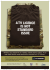### Discrete Math, Spring 2013 - Some Sample Problems### Semester I Examinations 2013-14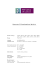### Untitled - Unfilodi### Transformers Alternators Optimus Prime Dodge 81327 Instructions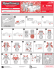### AUCTION INVENTORY REDUCTION &amp; MOVING Saturday, November 1 @ 10:00 AM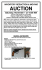### View Auction Flyer - Art Smith Auctioneers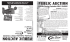### ENJJPT SC #70 T-6A Programs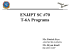### Modular Math - Walton High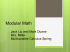### Transformers Masterpiece Rodimus Prime 32108 Instructions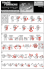### Official solutions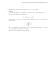### Extra Practice 1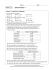### A Readable Introduction to Real Mathematics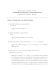### Assignment #3: Solutions - Applied Crypto Group at Stanford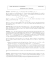### M = C d mod n.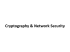### UOSEC Week 2: Asymmetric Cryptography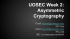### Solutions to 2015 CMO (DRAFTâas of April 6, 2015) Problem 1. Let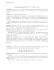### Math 1310 M1Z - Metropolitan Community College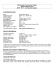### It’s Modern. It’s Modular. It’s the MOD* Dispensing System.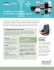### SQUARE PATTERNS, FERMAT NUMBERS, AND FIBONACCI NUMBERS 1. Introduction